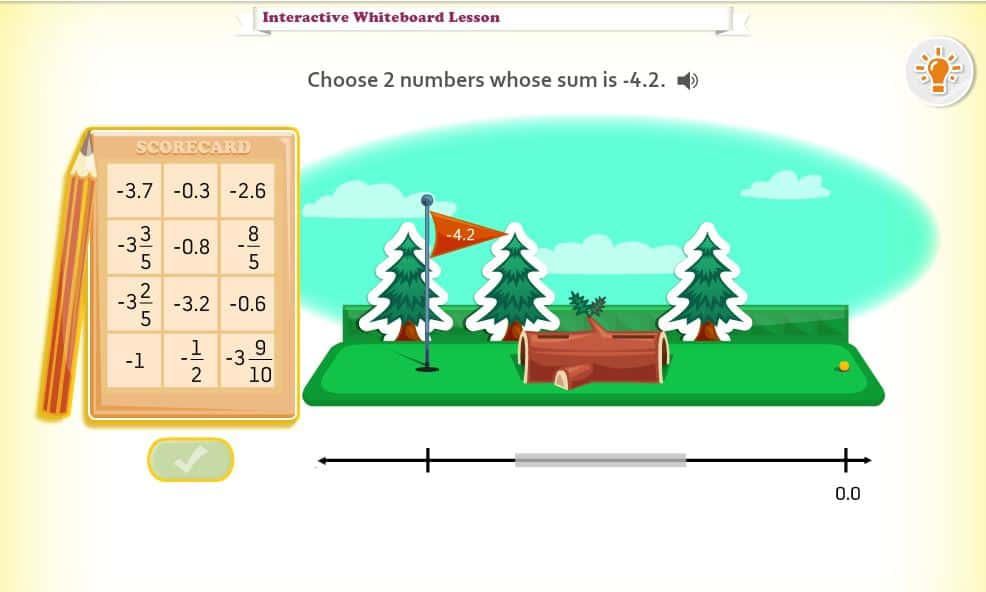Dream Box - Interactive Fraction and Decimal Sums. Develop fluency with addition & subtraction of fractions & decimals by choosing two numbers that have a target sum. Lesson

Dream Box - Interactive Subtracting Integers. Students use a number line and only the subtraction operation to solve for a variable in start, change, or result unknown situations. Lesson

Dream Box - Interactive Integer Operations.  Students use the order of operations to evaluate integer expressions involving addition, subtraction, multiplication and division. Lesson

CCSS.MATH.CONTENT.5.NF.A.1 (Somewhat)
Add and subtract fractions with unlike denominators (including mixed numbers) by replacing given fractions with equivalent fractions in such a way as to produce an equivalent sum or difference of fractions with like denominators. For example, 2/3 + 5/4 = 8/12 + 15/12 = 23/12. (In general, a/b + c/d = (ad + bc)/bd.)

CCSS.MATH.CONTENT.6.NS.B.3 (Somewhat)
Fluently add, subtract, multiply, and divide multi-digit decimals using the standard algorithm for each operation.

#### Apply and extend previous understandings of operations with fractions.

CCSS.MATH.CONTENT.7.NS.A.1
Apply and extend previous understandings of addition and subtraction to add and subtract rational numbers; represent addition and subtraction on a horizontal or vertical number line diagram.